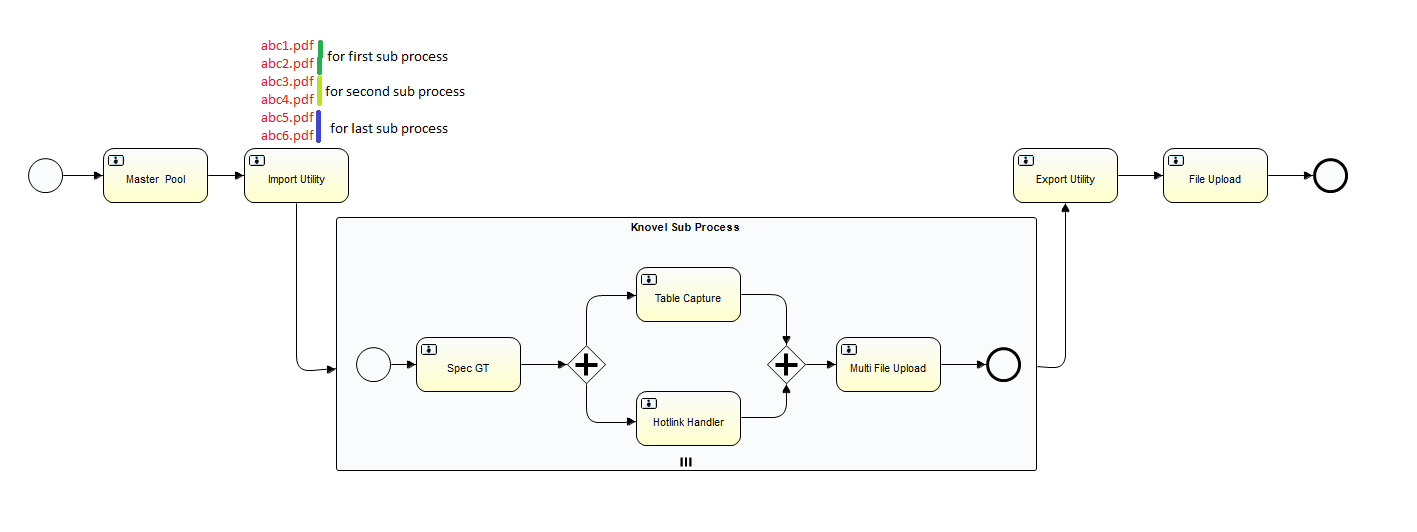## How to pass diffrent data value to Alfresco activiti multi instance sub process

cancel
Showing results for
Did you mean:Active Member II

## How to pass diffrent data value to Alfresco activiti multi instance sub process

I have created a multi instance sub process and the number of sub process is created dynamically using Multi-Instance's loopCardinality element but my problem is that I am not able to pass diffrent-diffrent data value to each sub process.

This is my problem scenario  as shown in the below image.I want to divide sub process based on loopCardinality value like:-

int getSubProcessDataValue(int fileCount,int  loopCardinality){

if(fileCount < 1 && loopCardinality < 1)

return 0

int result=fileCount/loopCardinality;

return result;

}

Suppose fileCount=7 and loopCardinality=2 then the above function will return 3 for first sub process.It means I have to pass 3 file names to first sub process.

int getLastSubProcessDataValue(int fileCount,int  loopCardinality){

if(fileCount < 1 && loopCardinality < 1)

return 0

int result=fileCount/loopCardinality;

int rem=fileCount%loopCardinality;

return result+rem;

}

Suppose fileCount=7 and loopCardinality=2 then the above function will return 4 for last sub process.It means I have to pass 4 file names to last sub process.1 Solution

Accepted SolutionsActive Member II

## Re: How to pass diffrent data value to Alfresco activiti multi instance sub process

I have done it using TaskListener as shown below code:

``package com.knovel.workflow.scripts;  import java.util.ArrayList; import java.util.Arrays; import java.util.List;  import org.activiti.engine.delegate.DelegateTask; import org.activiti.engine.delegate.TaskListener;  public class FileSplittingTaskListener implements TaskListener{  private static final long serialVersionUID = 3972525330472103945L;  @Override public void notify(DelegateTask task) {     System.out.println("#####FileSplittingTaskListener######");      task.setVariable("bpm_assignee", task.getVariable("bpm_assignee"));     task.setVariable("bpm_comment", task.getVariable("bpm_comment"));     task.setVariable("bpm_dueDate", task.getDueDate());     task.setVariable("bpm_priority", task.getPriority());      String strFileSplitter=(String)task.getVariable("wf_fileSplitter");     System.out.println("#############FileSplitter >>"+strFileSplitter);     Integer fileSplitter=Integer.parseInt(strFileSplitter);     System.out.println("#############FileSplitter >>"+fileSplitter);     //task.setVariable("wf_taskCounter", fileSplitter);      String workFlowFileName=(String)      task.getVariable("wf_workFlowFileName");     String[] files=workFlowFileName.split("-");     System.out.println("#######Files Length:"+files.length);     List<String[]> filesList = splitArray(files, fileSplitter);      List<String> fileList=new ArrayList<>();     for (String[] lists : filesList) {         String fileName="";         int srNo=0;         int count=1;         for (String string : lists) {             System.out.println("File>>"+string);             if(count == lists.length){                 fileName=fileName+ ++srNo +"-"+string;             }else{                 fileName=fileName+ ++srNo +"-"+string+",";             }             count++;         }         fileList.add(fileName);         srNo=0;      }      System.out.println("FileList>>"+fileList);      System.out.println("#############FileList >>"+fileList);      task.setVariable("filesList", fileList);      }      public static <T extends Object> List<T[]> splitArray(T[] array, int      max){     int x = array.length / max;     int r = (array.length % max); // remainder      int lower = 0;     int upper = 0;     List<T[]> list = new ArrayList<T[]>();     int i=0;      for(i=0; i<x; i++){        upper += max;        list.add(Arrays.copyOfRange(array, lower, upper));        lower = upper;      }       if(r > 0){        list.add(Arrays.copyOfRange(array, lower, (lower + r)));      }        return list;     }   }``

And I have updated multiInstanceLoopCharacteristics element properties as shown below:

``<multiInstanceLoopCharacteristics      isSequential="false"      activiti:collection="filesList"      activiti:elementVariable="wf_workFlowFileName"> </multiInstanceLoopCharacteristics>``

Thank you so much for your valuable supports!!!

2 RepliesActive Member II

## Re: How to pass diffrent data value to Alfresco activiti multi instance sub process

I have done it using TaskListener as shown below code:

``package com.knovel.workflow.scripts;  import java.util.ArrayList; import java.util.Arrays; import java.util.List;  import org.activiti.engine.delegate.DelegateTask; import org.activiti.engine.delegate.TaskListener;  public class FileSplittingTaskListener implements TaskListener{  private static final long serialVersionUID = 3972525330472103945L;  @Override public void notify(DelegateTask task) {     System.out.println("#####FileSplittingTaskListener######");      task.setVariable("bpm_assignee", task.getVariable("bpm_assignee"));     task.setVariable("bpm_comment", task.getVariable("bpm_comment"));     task.setVariable("bpm_dueDate", task.getDueDate());     task.setVariable("bpm_priority", task.getPriority());      String strFileSplitter=(String)task.getVariable("wf_fileSplitter");     System.out.println("#############FileSplitter >>"+strFileSplitter);     Integer fileSplitter=Integer.parseInt(strFileSplitter);     System.out.println("#############FileSplitter >>"+fileSplitter);     //task.setVariable("wf_taskCounter", fileSplitter);      String workFlowFileName=(String)      task.getVariable("wf_workFlowFileName");     String[] files=workFlowFileName.split("-");     System.out.println("#######Files Length:"+files.length);     List<String[]> filesList = splitArray(files, fileSplitter);      List<String> fileList=new ArrayList<>();     for (String[] lists : filesList) {         String fileName="";         int srNo=0;         int count=1;         for (String string : lists) {             System.out.println("File>>"+string);             if(count == lists.length){                 fileName=fileName+ ++srNo +"-"+string;             }else{                 fileName=fileName+ ++srNo +"-"+string+",";             }             count++;         }         fileList.add(fileName);         srNo=0;      }      System.out.println("FileList>>"+fileList);      System.out.println("#############FileList >>"+fileList);      task.setVariable("filesList", fileList);      }      public static <T extends Object> List<T[]> splitArray(T[] array, int      max){     int x = array.length / max;     int r = (array.length % max); // remainder      int lower = 0;     int upper = 0;     List<T[]> list = new ArrayList<T[]>();     int i=0;      for(i=0; i<x; i++){        upper += max;        list.add(Arrays.copyOfRange(array, lower, upper));        lower = upper;      }       if(r > 0){        list.add(Arrays.copyOfRange(array, lower, (lower + r)));      }        return list;     }   }``

And I have updated multiInstanceLoopCharacteristics element properties as shown below:

``<multiInstanceLoopCharacteristics      isSequential="false"      activiti:collection="filesList"      activiti:elementVariable="wf_workFlowFileName"> </multiInstanceLoopCharacteristics>``

Thank you so much for your valuable supports!!!Senior Member